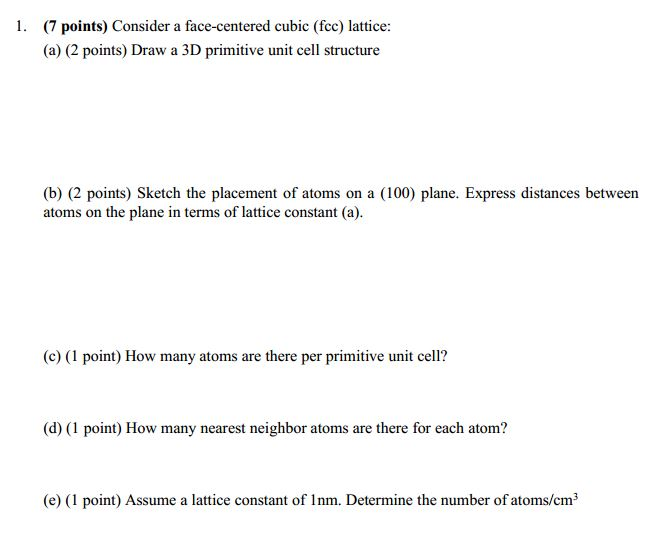Home / Answered Questions / Other / 1-7-points-consider-a-face-centered-cubic-fcc-lattice-a-2-points-draw-a-3d-primitive-unit-cell-struc-aw472

# (Solved): 1. (7 Points) Consider A Face-centered Cubic (fcc) Lattice: (a) (2 Points) Draw A 3D Primitive Unit ...1. (7 points) Consider a face-centered cubic (fcc) lattice: (a) (2 points) Draw a 3D primitive unit cell structure (b) (2 points) Sketch the placement of atoms on a (100) plane. Express distances between atoms on the plane in terms of lattice constant (a). (c) (1 point) How many atoms are there per primitive unit cell? (d) (1 point) How many nearest neighbor atoms are there for each atom? (e) (1 point) Assume a lattice constant of Inm. Determine the number of atoms/em

We have an Answer from Expert